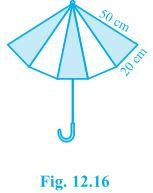# An umbrella is made by stitching 10 triangular pieces of cloth of two different colours (see Fig.12.16) , each piece measuring 20 cm, 50 cm and 50 cm. How much cloth of each colour is required for the umbrella?Solution

Using Heron’s formula, area of the triangle =$$\sqrt{s(s-a)(s-b)(s-c)}$$

Each ar(△)can be calculated by Heron’s Formula, where

a = 50 cm

b = 50 cm

c = 20 cm

Semi Perimeter (s) = a+b+c/2

s = 50+50+20/2

s = 60 cm

ar(△) = √s(s-a)(s-b)(s-c)

= √60(60-50)(60-50)(60-20)

= √60×(10)×(10)×(40)

= 200√6 cm2

Hence, the Total Area = 5×200√6

= 1000√6 cm2

Total Area 1000√6 cm2